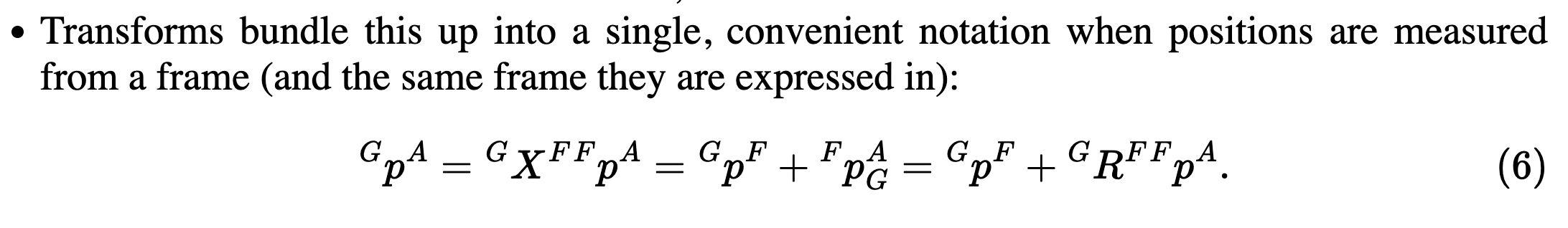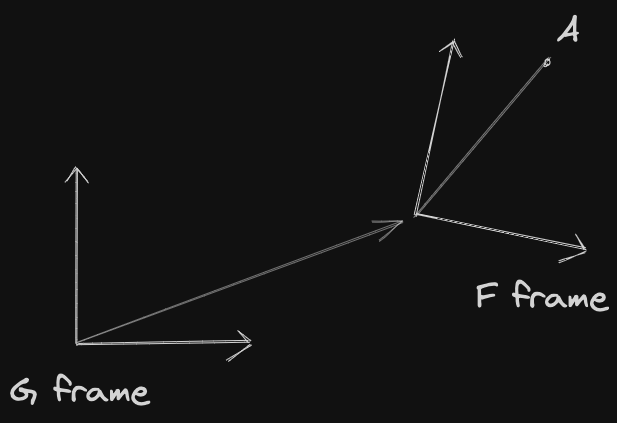Rigid-Body Transformations

# Spatial Algebra / Euclidean Geometry §

This is the technical page for Rigid-Body Transformations. We implement all of this with SE(3) matrices ( matrix).

First seriously learned from the MIT Manipulation course. See Drake (Robot) for notation. Notes from https://manipulation.mit.edu/pick.html#spatial_algebra and Visual SLAM book.

On a day to day, I feel like you really don’t need to complicate yourself with these notations, something like this is enough.

High Level

Calculate the point in reference frame when you are given in reference frame :

Calculate the orientation

• Note that even though these operations are not commutative, it works because rotation matrices are orthogonal, i.e.

Being very familiar with Matrix Transformation is super helpful.

### Theory from MIT §

If you want to get the position of measured from frame (frame of the gripper), but you measure the position of from frame , you can do so by multiplying the point in frame by the transform between the two frames

• The really important thing not to confuse is that you use transform , and not . it takes a bit of time to do some convincing to myself, draw it outNow, how is this specifically represented? Is a matrix? How does it work?

• So the transform includes both translation and rotation. Translation is a vector since we simply add a 3D point. The rotation depends on what representation, usually a rotation matrix
• HOWEVER, we can actually represent as a matrix, using the Special Euclidean Group, so that rotation and translation are stored in the same matrix!! And then, you can just multiply matrices together, and write as a vector. More details below

I think I got it!!

Rotation has many common representations, including

Why all these representations? The main issue is Gimbal Lock.

Why all of the representations?

Shouldn’t “roll-pitch-yaw” be enough? Unfortunately, no. The limitation is perhaps most easily seen by looking at the coordinate changes from roll-pitch-yaw to/from a rotation matrix. Any roll-pitch-yaw can be converted to a rotation matrix, but the inverse map has a singularity. In particular, when the pitch angle is , then roll and yaw are now indistinguishable. The physical manifestation of this phenomenon is called Gimbal Lock, where we lose a DOF.

Similarly, the direction of the vector in the axis-angle representation in not uniquely defined when the rotation angle is zero. These singularities become problematic when we start taking derivatives of rotations, for instance when we write the equations of motion. It is now well understood that it requires at least four numbers to properly represent the group of 3D rotations; the unit quaternion is the most common four-element representation.

Also, another issue is like in this case, where you roll first or pitch first produces different output points.

Why don't these issues arise with 2D representations?

Because in 2D, rotation is only around a single axis (the z-axis, the one that comes out of the page towards your face).

Also see

### Composition of Transforms using SE(3) (Euclidean Transforms) §

I was asked this at my NVIDIA interview. I should have known this, but now I know. This is learned from the Visual SLAM book, page 36.

We need to use Homogeneous Coordinates.

This is slightly different notation than above, because I took it from a different book. At this point, the coordinate frames of each is implied, you should know.

Suppose we made two transformations, and . The transform from to is

This is not a super elegant form after multiple transformations. We can introduce Homogeneous Coordinates by simply adding 1 at the end of the 3D vector. Then, we can apply the Rotation and Translation Matrix using the same matrix!!!

\left[\begin{array}{l} \mathbf {a} ' \\ 1 \end{array} \right] = \left[ {\begin{array}{*{20}{c}} \mathbf{R}&\mathbf{t}\\ {{\mathbf{0}^T}}&1 \end{array}} \right] \left[ \begin{array}{l} \mathbf {a} \\ 1 \end{array} \right] \buildrel \Delta \over = \mathbf{T} \left[ \begin{array}{l} \mathbf {a} \\ 1 \end{array} \right] \end{equation}

Why does this work?

Just write down the matrix multiplication. You’ll see that the first row is , while the second row is , so everything works out!

The transformation matrix is called a Special Euclidean Group .

\begin{equation} \mathrm{SE}(3) = \left\{ \mathbf{T} = \left[ {\begin{array}{*{20}{c}} \mathbf{R} & \mathbf{t} \\ {{\mathbf{0}^T}} & 1 \end{array}} \right] \in \mathbb{R}^{4 \times 4} | \mathbf{R} \in \mathrm{SO}(3), \mathbf{t} \in \mathbb{R}^3\right\} \end{equation}

We temporarily use to represent the homogeneous coordinates of . Then, relying on homogeneous coordinates and transformation matrices, the superposition of the two transformations can have a good form:

Back to the Drake (Robot) notation, this is how you can compose transforms by having

### Implementation in Eigen using Isometry3d §

To use the Euclidean Transformation in Eigen, we use Isometry3d. There are several ways to initialize:

Isometry3d T = Isometry3d::Identity();
Isometry3d T(q); // IMPORTANT: q must be a UNIT quaterion
Isometry3d T(rotation_matrix);
Isometry3d T; // garbage values

Rotation and translation

// MAKE SURE T is intialized to Identity()
T.rotate(rotation_vector); // Accepts Quateriond, AngleAxisd, or Matrix3d
T.pretranslate(Vector3d(1,3,4));
/*
Tranform matrix =
0.707 -0.707      0      1
0.707  0.707      0      3
0      0      1      4
0      0      0      1
*/
• IMPORTANT: Under the hood, what actually is happening is doing a matrix multiplication, instead of writing to the entries, which is slower

To apply the transformation, we simply multiply

Vector3d v_transformed = T * v; // Equivalent to R * v + t

Composition of transformations

Isometry3d T1;
Isometry3d T2;

// Set T1 and T2 to correct values
...

// Then, composition of transforms is done like this
T2 * T1 * Vector3d(1,2,3);

Other things

T.rotation(); // extract rotation matrix (3x3)
T.translation(); // extract translation vector (3x1)

Question pretranslate vs translate?

pretranslate and translate differ in whether they apply the argument from the right or from the left

Calling pretranslate() applies B on the from the left, returning B*A:

A.pretranslate(B)

Calling translate()applies it from the right, returning A*B:

A.translate(B)

Source: Stackoverflow

This distinction is SUPER important.

• Actually, we work work with these transformations (rotation and translation), everything is done through matrix multiplication of matrices. Yes, even translation. This makes the code run a lot faster.

Rotate, then Translate

I heard this before but was not convinced. This stackexchange post really helps.

Remember that when you call translate(), this is actually implemented through a matrix multiplication. If you then call rotate(), this calls rotate on the translated point, which would be INCORRECT.

Let’s look at the math real quick:

Correct: Incorrect:

So when you have , in my mind, I’m “translating first, then rotating”, but no, you are applying the rotation first to the point, then translating. Also see Change of Basis.

Rule of Thumb

My rule of thumb is to always use pretranslate and rotate as I learned from the Visual SLAM book. Only use these functions to set the initial values of the Isometry3d matrix. Then, composition of transforms should be done by doing something like T2 * T1.

• Ahh I see why they do this. If you always call pretranslate and rotate, then you are less prone to mistakes. This is clearer with the example below
• If you do prerotate and pretranslate, you are prone to mistakes. You need to call prerotate first

Example where it breaks down if you don’t respect this:

Isometry3d T = Isometry3d::Identity();
Vector3d translation(2,5,5);
Matrix3d rotation_matrix = Quaterniond(1, 0.2,0.4,0.2).toRotationMatrix();
/*
0.6 -0.24  0.88
0.56  0.84 -0.24
-0.72  0.56   0.6
*/

// GOOD
T.rotate(rotation_matrix);
T.pretranslate(translation);
/* T=
0.6 -0.24  0.88     2
0.56  0.84 -0.24     5
-0.72  0.56   0.6     5
0     0     0     1
*/

Isometry3d T2 = Isometry3d::Identity();
T2.prerotate(rotation_matrix);
T2.translate(translation);
/*
0.6 -0.24  0.88   4.4
0.56  0.84 -0.24  4.12
-0.72  0.56   0.6  4.36
0     0     0     1
*/

// WORKS too, but You gotta BE SUPER CAREFUL TO CALL ROTATION FIRST
Isometry3d T3 = Isometry3d::Identity();
T3.prerotate(rotation_matrix);
T3.pretranslate(translation);
/* T=
0.6 -0.24  0.88     2
0.56  0.84 -0.24     5
-0.72  0.56   0.6     5
0     0     0     1
*/
// WORKS, don't need to worry about which to call first
Isometry3d T4 = Isometry3d::Identity();
T4.pretranslate(translation);
T4.rotate(rotation_matrix);

See clarification under Change of Basis.

#### Composition of Rotation §

You can just multiple the rotation matrices together. See Rotation Matrix.